#Calculus Worksheets

## Differentiation Rules Worksheets

Here is a graphic preview for all of the Differentiation Rules for Calculus Worksheets. You can select different variables to customize these Differentiation Rules for Calculus Worksheets for your needs. The Differentiation Rules for Calculus Worksheets are randomly created and will never repeat so you have an endless supply of quality Differentiation Rules for Calculus Worksheets to use in the classroom or at home. We have differentiation tables, rate of change, product rule, quotient rule, chain rule, and derivatives of inverse functions worksheets.

Our Differentiation Rules for Calculus Worksheets are free to download, easy to use, and very flexible.

These Differentiation Rules for Calculus Worksheets are a good resource for students in high school.

Click here for a Detailed Description of all the Differentiation Rules for Calculus Worksheets.

## Quick Link for All Differentiation Rules for Calculus Worksheets

Click the image to be taken to that Differentiation Rules for Calculus Worksheets.

##### Differentiation Tables Worksheets##### Average Rate of Change Worksheets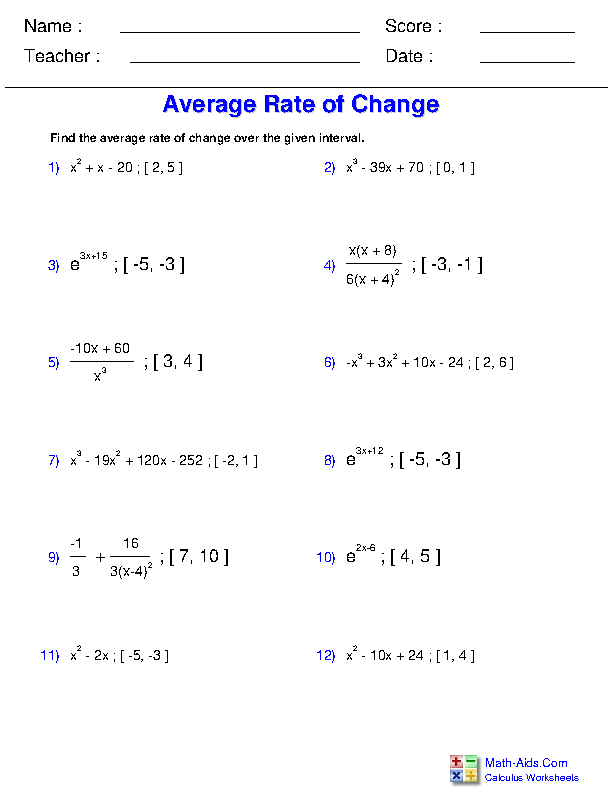##### Definition of the Derivative Worksheets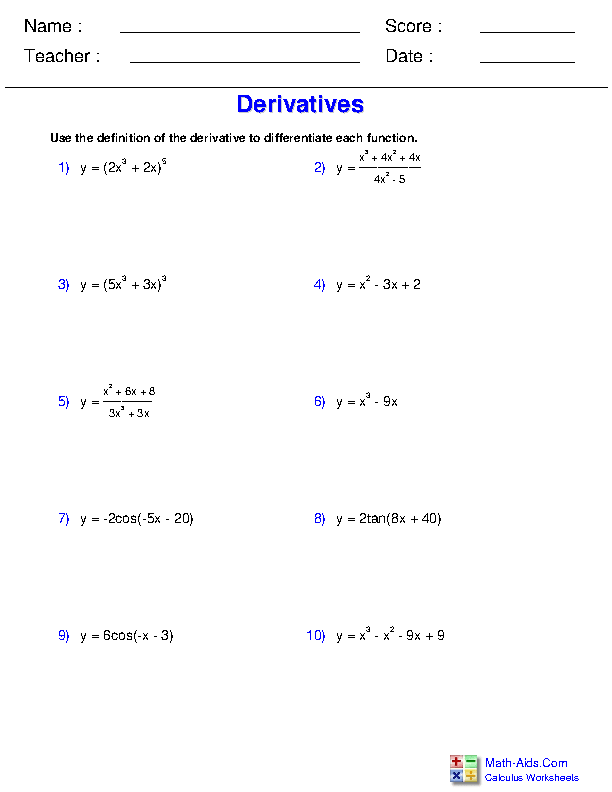##### Power, Constant, and Sum Rules Worksheets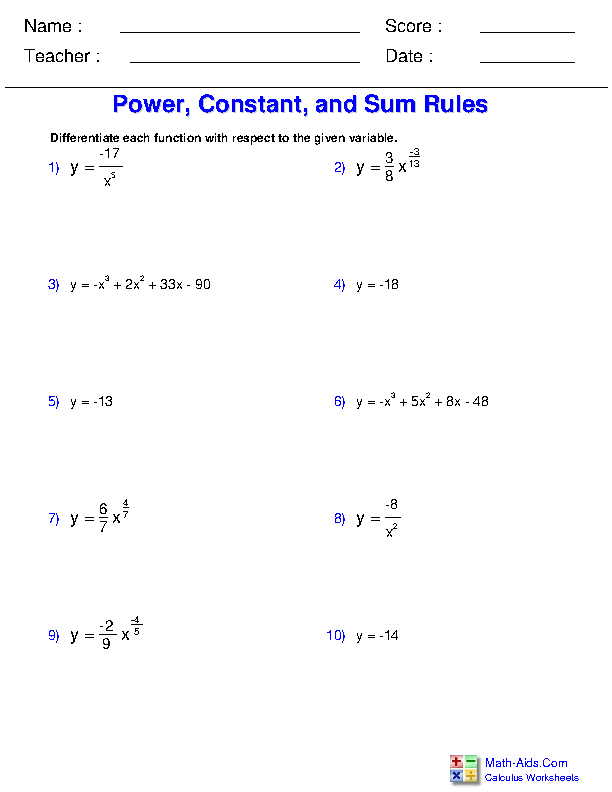##### Product Rule Worksheets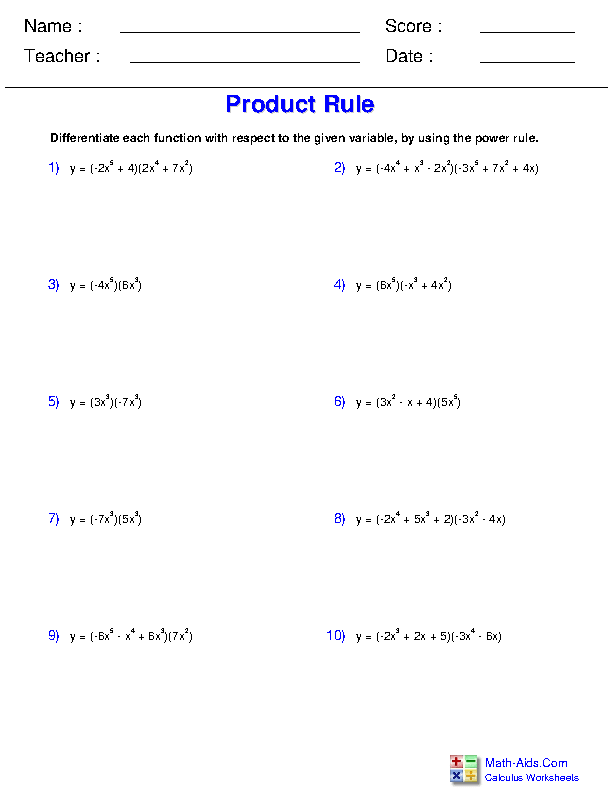##### Quotient Rule Worksheets##### Chain Rule Worksheets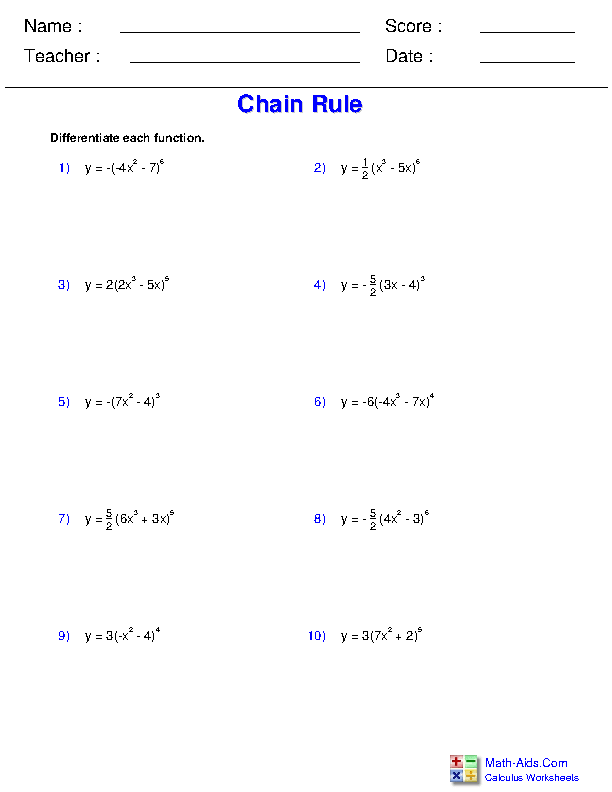##### Chain Rule with Trigonometry Worksheets##### Chain Rule with Inverse Trigonometric Functions Worksheets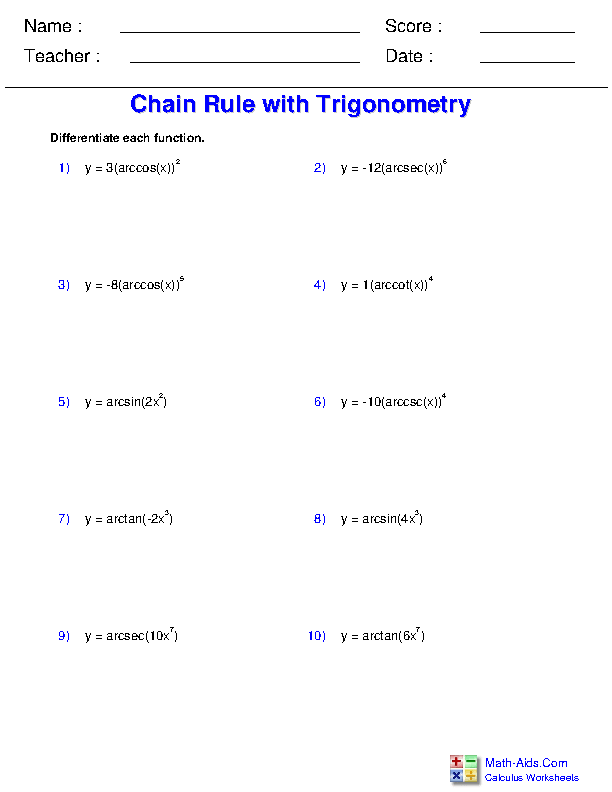##### Higher Order Derivatives Worksheets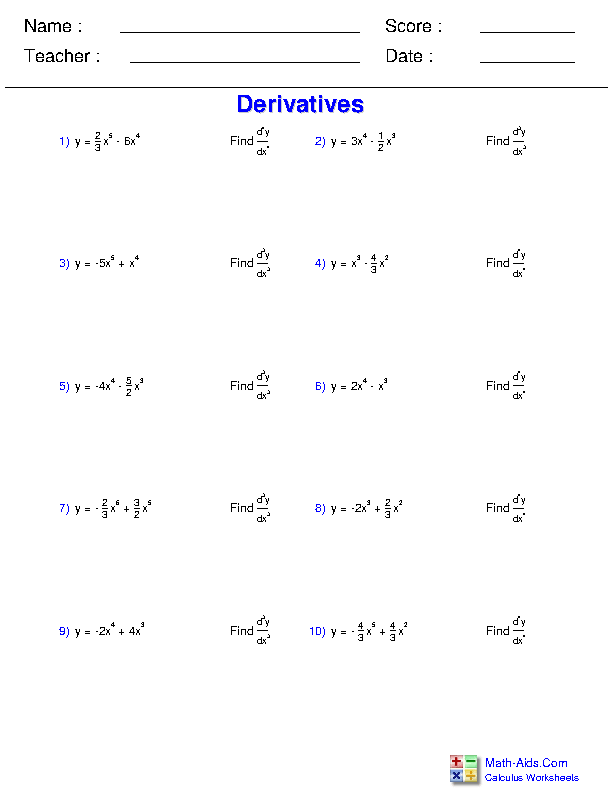##### Implicit Differentiation Worksheets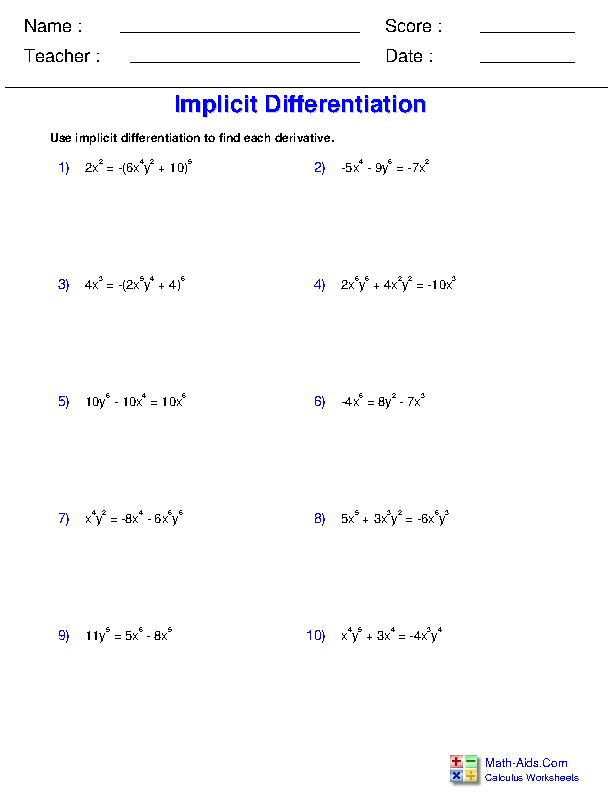##### Instantaneous and Average Rate of Change Worksheets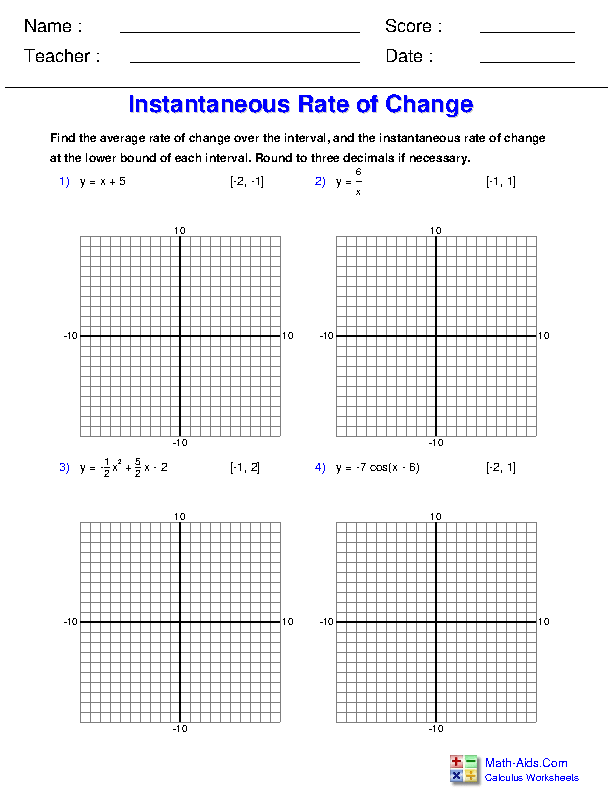##### Logarithmic Differentiation Worksheets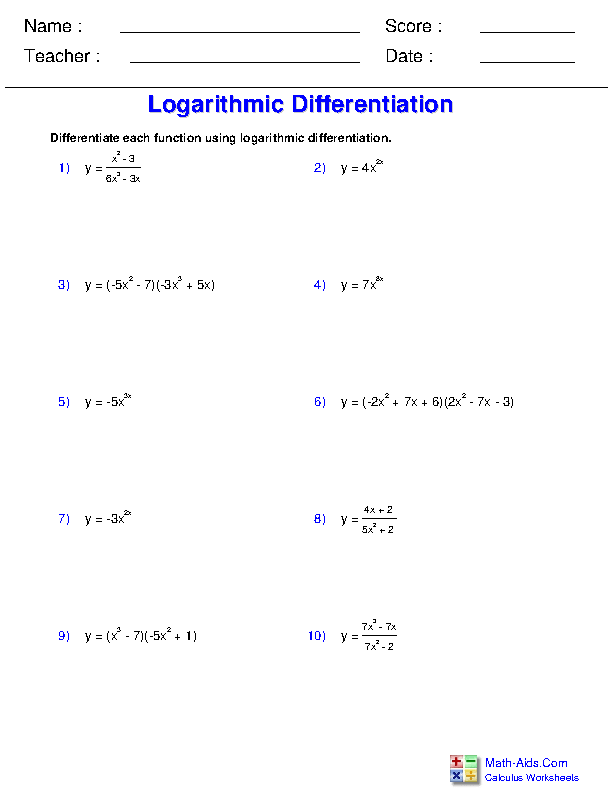##### Derivatives of Inverse Functions by Direct Computation Worksheets##### Derivatives of Inverse Functions Worksheets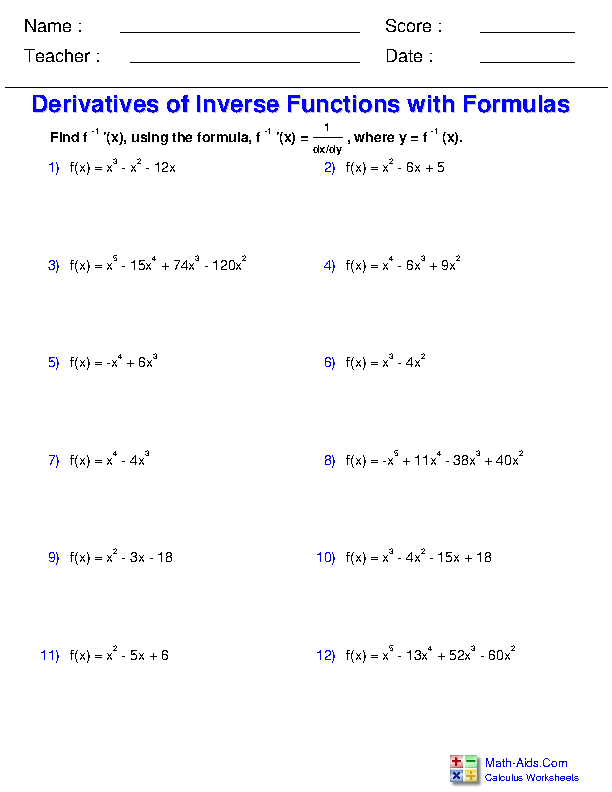Recommended Videos

## Detailed Description for All Differentiation Rules for Calculus Worksheets

Differentiation Tables Worksheets
This Calculus - Differentiation Rules Worksheet will produce problems that involve using functions tables to understand rules of differentiation.

Average Rate of Change Worksheets
This Calculus - Differentiation Rules Worksheet will produce problems that involve finding the average rate of change of a function.

Definition of the Derivative Worksheets
This Calculus - Differentiation Rules Worksheet will produce problems that deal with using the definition of the derivative to solve problems.

Power, Constant, and Sum Rules Worksheets
This Calculus - Differentiation Rules Worksheet will produce problems that involve using the power, constant and sum rules of differentiation.

Product Rule Worksheets
This Calculus - Differentiation Rules Worksheet will produce problems that involve using product rule of differentiation.

Quotient Rule Worksheets
This Calculus - Differentiation Rules Worksheet will produce problems that involve using the quotient rule to differentiate functions.

Chain Rule Worksheets
This Calculus - Differentiation Rules Worksheet will produce problems that involve using the chain rule to differentiate functions.

Chain Rule with Trigonometry Worksheets
This Calculus - Differentiation Rules Worksheet will produce problems that involve using the chain rule to differentiate functions that include trigonometry.

Chain Rule with Inverse Trigonometric Functions Worksheets
This Calculus - Differentiation Rules Worksheet will produce problems that involve using the chain rule to differentiate inverse trigonometric functions.

Higher Order Derivatives Worksheets
This Calculus - Differentiation Rules Worksheet will produce problems that deal with solving for higher order derivatives.

Implicit Differentiation Worksheets
This Calculus - Differentiation Rules Worksheet will produce problems that involve implicit differentiation.

Instantaneous and Average Rate of Change Worksheets
This Calculus - Differentiation Rules Worksheet will produce problems that deal with finding the instantaneous and average rate of change over an interval for a function.

Logarithmic Differentiation Worksheets
This Calculus - Differentiation Rules Worksheet will produce problems that involve logarithmic differentiation.

Derivatives of Inverse Functions by Direct Computation Worksheets
This Calculus - Differentiation Rules Worksheet will produce problems that involve finding the derivatives of inverse functions by direct computation.

Derivatives of Inverse Functions Worksheets
This Calculus - Differentiation Rules Worksheet will produce problems that involve finding the derivatives of inverse functions.